# Internal Division

Internal Division

## Introduction:

In this section, we are going to explore how to divide internally the given line segment in a given ratio by construction. Also, we are going to use the Basic Proportionality Theorem which states that "If a line is drawn parallel to one side of a triangle to intersect the other two sides in distinct points, the other two sides are divided in the same ratio".

## Internal Division:

You are given a line segment $$AB$$: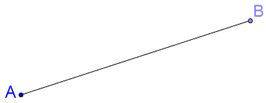How will you divide it into a given (rational) ratio? Suppose that you are asked to internally divide $$AB$$ in the ratio $$3:4$$ . This means that you have to find a point $$C$$ on $$AB$$ such that $$AC:CB = 3:4$$ , as shown below: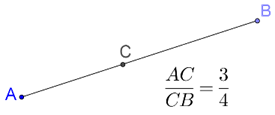How will you geometrically locate point $$C$$? This is the problem of the internal division of a given line segment in a given ratio. We are going to use BPT to guide our construction.

## Solved Example:

Example 1: Divide $$AB$$ of any length in the ratio $$3:4$$ internally.

Solution: Let us follow the steps of construction as given below:

Steps of construction for internal division:

Step 1: Draw a line segment $$AB$$ of any length.

Step 2: Draw any ray $$AX$$ inclined at an arbitrary angle to $$AB$$ , and mark $$3 + 4 = 7$$ equal intervals on $$AX$$ , as shown below: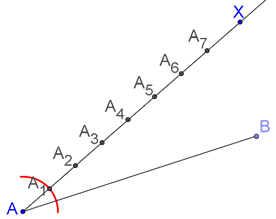This can be done easily using a compass. In the figure, we have shown one arc (to construct the first interval)

Step 3: Join the last point (in this case, $${A_7}$$ ) to B: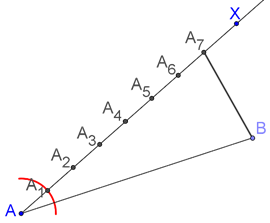Step 4: Through $${A_3}$$ , draw a parallel to $${A_7}B$$ . The point of intersection of this parallel with $$AB$$ is the required point $$C$$: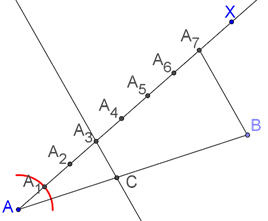The proof is straightforward.

We have $${A_3}C\parallel {A_7}B$$ . Applying BPT in $$\Delta AB{A_7}$$ , we have:

$\frac{{A{A_3}}}{{{A_3}{A_7}}} = \frac{{AC}}{{CB}}$

$\Rightarrow \boxed{\frac{{AC}}{{CB}} = \frac{3}{4}}$

Note: Using this approach, we can divide any given segment in an arbitrary rational ratio.Challenge: Construct some line segments and divide them into the following ratios:

$3:5,{\text{ }}2:3,{\text{ }}1:7,{\text{ }}6:7,{\text{ }}2:11$

⚡Tip: Use the same steps of construction as we have used in the above example.

Similar Triangles
Similar Triangles
grade 9 | Questions Set 2
Similar Triangles
Similar Triangles
grade 9 | Questions Set 1
More Important Topics
Numbers
Algebra
Geometry
Measurement
Money
Data
Trigonometry
Calculus
More Important Topics
Numbers
Algebra
Geometry
Measurement
Money
Data
Trigonometry
Calculus